Post update: Saturday May 21st, 2016
This post was originally written two years ago and admittedly took an angle that downplayed the importance of automaticity of multiplication facts which was not the original intention. Please read on as I attempt to clarify the original intent of the article.

## Let’s Promote Understanding to Build Automaticity Over Time

As you may have heard in the Globe and Mail or in my recent post, Ontario Education Minister, Liz Sandals recently tossed a comment into the media about the need for students to know their math facts:

That’s actually a great homework assignment: Learn your multiplication tables.

Liz Sandals – Ontario Minister of Education

## Understanding vs. Memorizing Multiplication Tables

It seems that whenever things aren’t going well in the world of math education – or more poorly than usual – people are quick to claim that it is because students don’t know their basic math facts; namely, memorizing multiplication tables. This can be problematic for children as they grow older, multiplication will be widely used when they’re older and working. To help your children understand multiplication from a young age, you might want to consider giving them extra multiplication worksheets outside of school to ensure they understand the skill. It’s important that children understand this type of maths because there is an obvious benefit to knowing your multiplication tables when solving problems, but is it only the memorization of basic math that students lack?

If we recall what memorizing multiplication tables looked like when we were in school, you might picture flash cards, repeating products aloud or writing out your 7’s times tables repeatedly until it was engrained in your mind. There is no doubt in my mind that having multiplication tables memorized allows for making calculations without taxing working memory.

## Memorizing Math Does Not Equal Understanding

Although the debate between traditional and reformed mathematics has been going on for decades, I think both groups ultimately want the same thing: students to be proficient in mathematics. However when people make statements about memorizing math facts or “going back to the basics”, people likely have very different interpretations as to how we should get there.

There are no silver bullets in math education and the memorization of multiplication tables alone will not solve all of the problems our students face. That said, many suggest that Ontario needs to “go back to the basics”, but did they ever leave? While the memorization of multiplication tables is not explicitly stated in the Ontario math curriculum, there is a huge push to develop a deep understanding of what it really means to multiply and in time, this should promote automaticity. As I mentioned in this post, shortcuts in math are only effective when you know how to take the long way; learning multiplication is no exception.

Check out the wording of an overall expectation in the Grade 2 Number Sense and Numeration Strand of the curriculum:

solve problems involving the addition and subtraction of one- and two-digit whole numbers using a variety of strategies, and investigate multiplication and division.

We could easily change that overall expectation to say:

know multiplication tables up to 5 times 5 without a calculator.

Which one would be more valuable to the student?

The grade 1-8 Ontario math curriculum contains the word multiplication a total of 47 times, which hardly suggests that multiplication is not a focus throughout elementary.

## A Variety of…

The curriculum doesn’t suggest that students should not know their multiplication tables, but instead has a greater focus on developing multiplicative skills and understanding. Digging deeper into the document, the words “variety of” occurs in the curriculum a total of 179 times and suggests that much more attention should be spent on exposing students to a variety of strategies, using a variety of tools, to show understanding a variety of ways. If done well, I would have to believe students would have a very deep understanding of math concepts including multiplication.

Over time, I’d like to think that an effective implementation of the Ontario Grade 1-8 Math Curriculum would allow for committing multiplication tables to memory over time.

## So What’s the Problem?

The curriculum document doesn’t explicitly state the memorization of multiplication tables as an expectation, but it does require that concepts be delivered using a variety of strategies and tools/manipulatives. Where the problem may lie is how the curriculum expectations around multiplication are interpreted by the teacher.

What if the teacher isn’t comfortable teaching math? What if they only really understand one way to multiply? Does “a variety” mean three strategies? Four strategies? … Ten?

These are just a few of the questions that pop into my head when I read the curriculum and ponder some of the challenges we continue to experience in mathematics education.

## Case Study

Tom is a teacher, who admits not having a real passion for teaching math. When he teaches multiplication, it might look like this:

### Multiplication Table: 3 x 2 = 6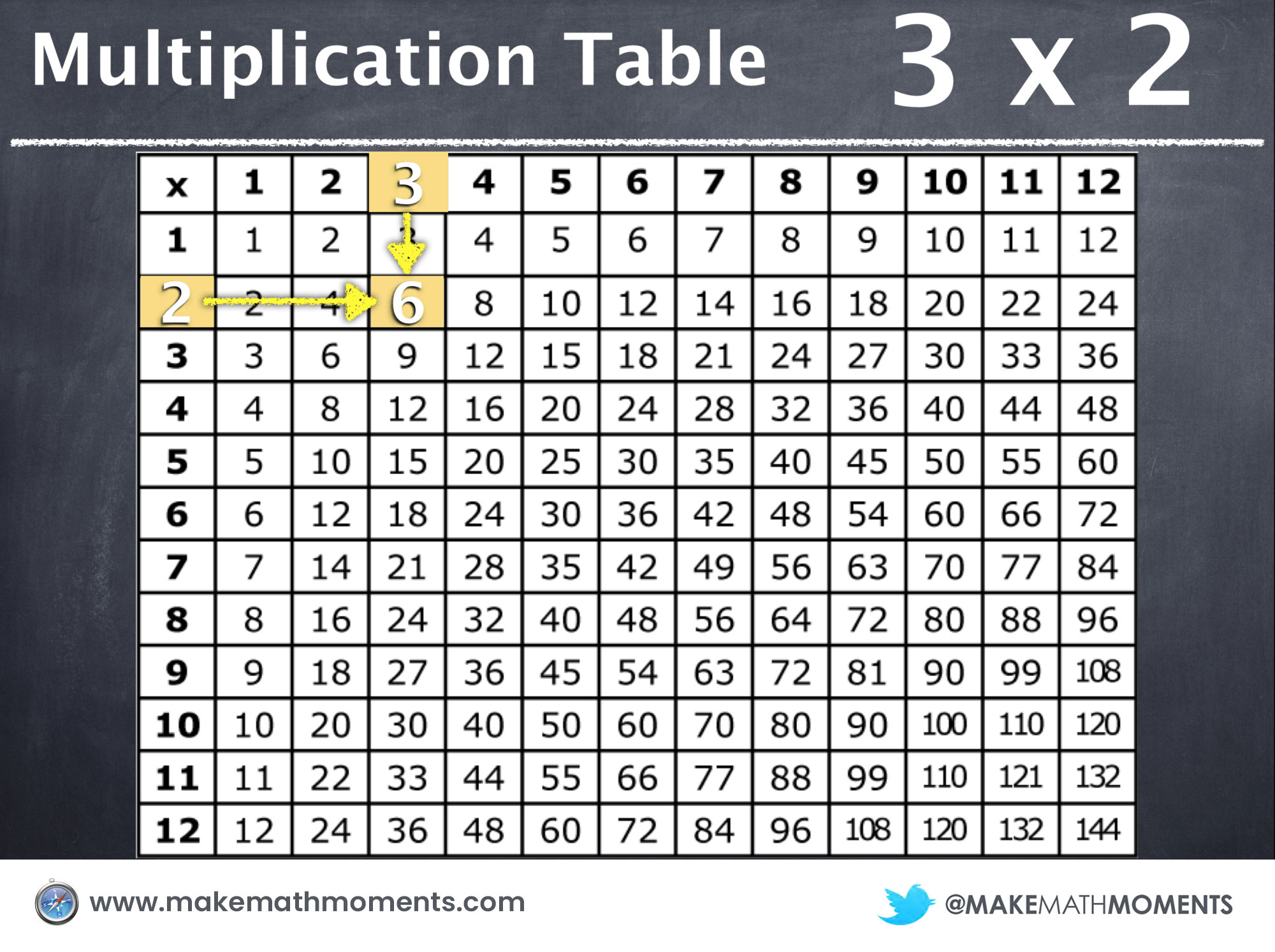Great for patterns and provides a strategy for students to “get there” if they are stuck, but might not be enough for students to build a deep conceptual understanding.

### Multiplication Algorithm: 12 x 9 = 108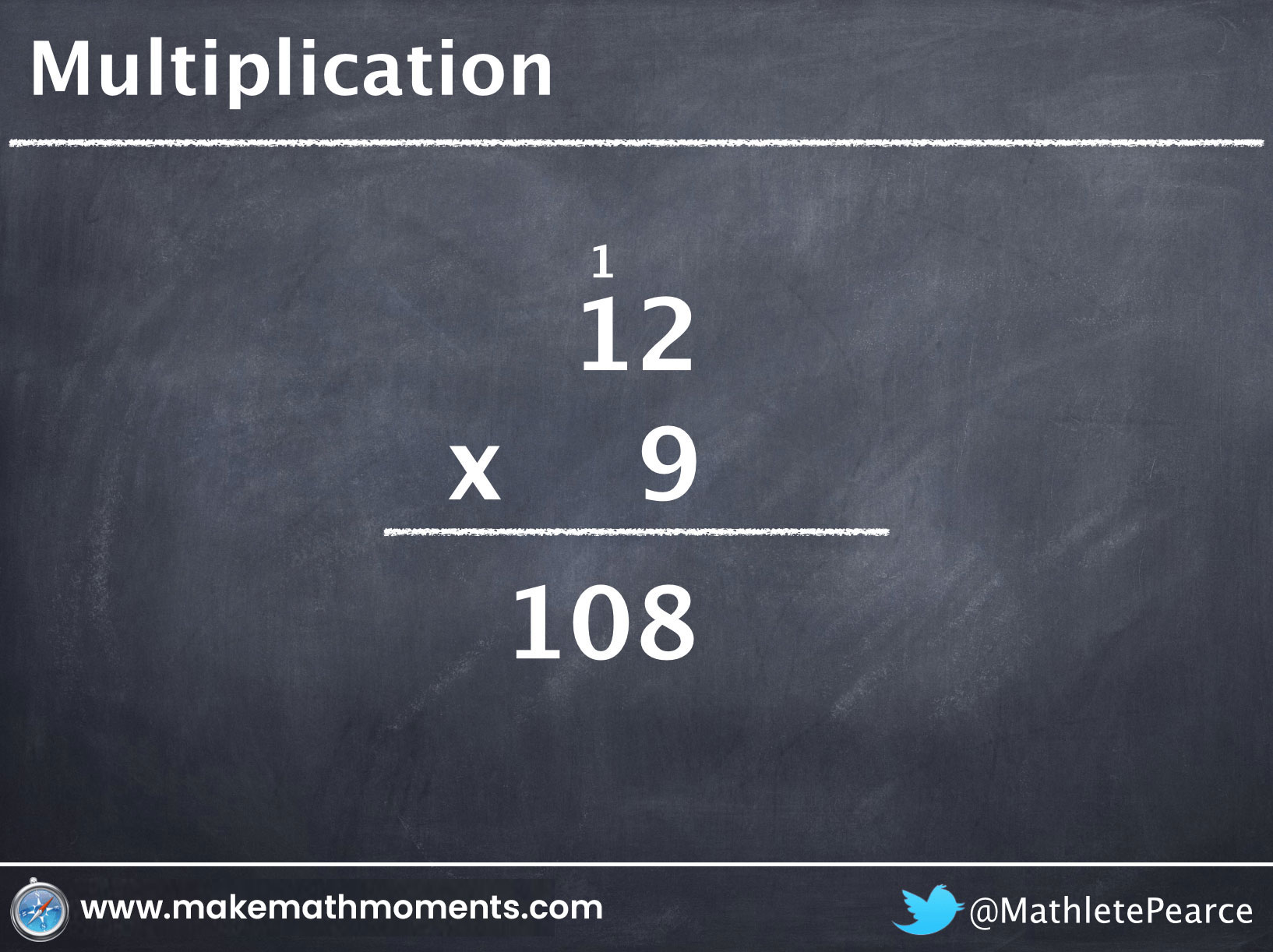We all know the algorithm and it is likely that teachers will use it moving forward as students begin working with larger numbers. Works like a charm if you do each step correctly. Something very interesting about the algorithm is that it can often be taught as a procedure and nothing more. Knowing how to use the algorithm without a conceptual understanding of how it works can be useful, but possibly only slightly more useful than typing the expression into a calculator. Miss a step or press the wrong button without a deep understanding of how the algorithm works and you might be out of luck.

Rather than debating over whether memorizing multiplication tables is necessary, maybe a better question might be:

What is the most effective way to have our students build automaticity with multiplication and other math facts?

## Visualizing Multiplication

Can we promote the automaticity of multiplication facts by building a deep conceptual understanding and spacing the practice meaningfully throughout our math curriculum? Would helping students visualize how multiplication works allow for this memorization to build over time?

Here are just a few visual strategies that might help students build a deeper conceptual understanding than simply learning their times tables through mass practice.

## Basic Multiplication As An Array: 3 x 2 = 3 groups of 2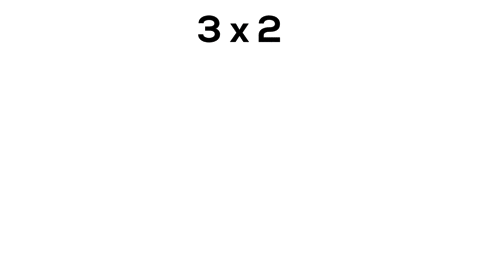### Multiplication By Chunking: 12 x 9 = 3(3) + 3(4)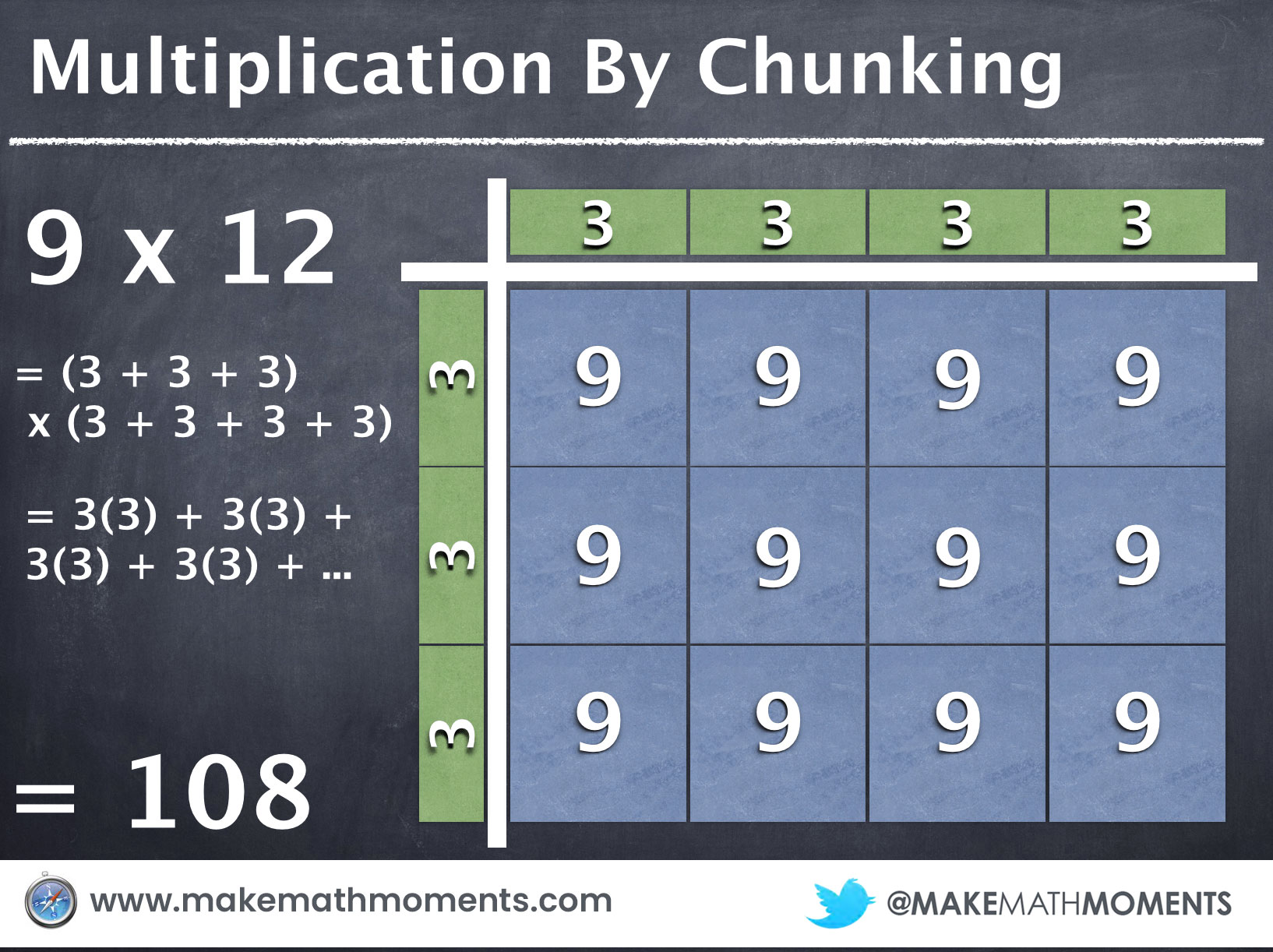Chunking through the use of an area model is a skill that can be used not only to lower the bar for all learners at every level of readiness to begin multiplying with confidence.

If we promote the use of multiple strategies to complete mental math rather than turning to the calculator, then I believe students will value the skill of multiplication automaticity and feel the need to use it as an efficient strategy. I must be clear in saying that this will not happen unless we promote the use of mental math and multiplication strategies throughout the entirety of our math courses.

### Multiplication By Chunking: 12 x 9 = 9(10) + 9(2)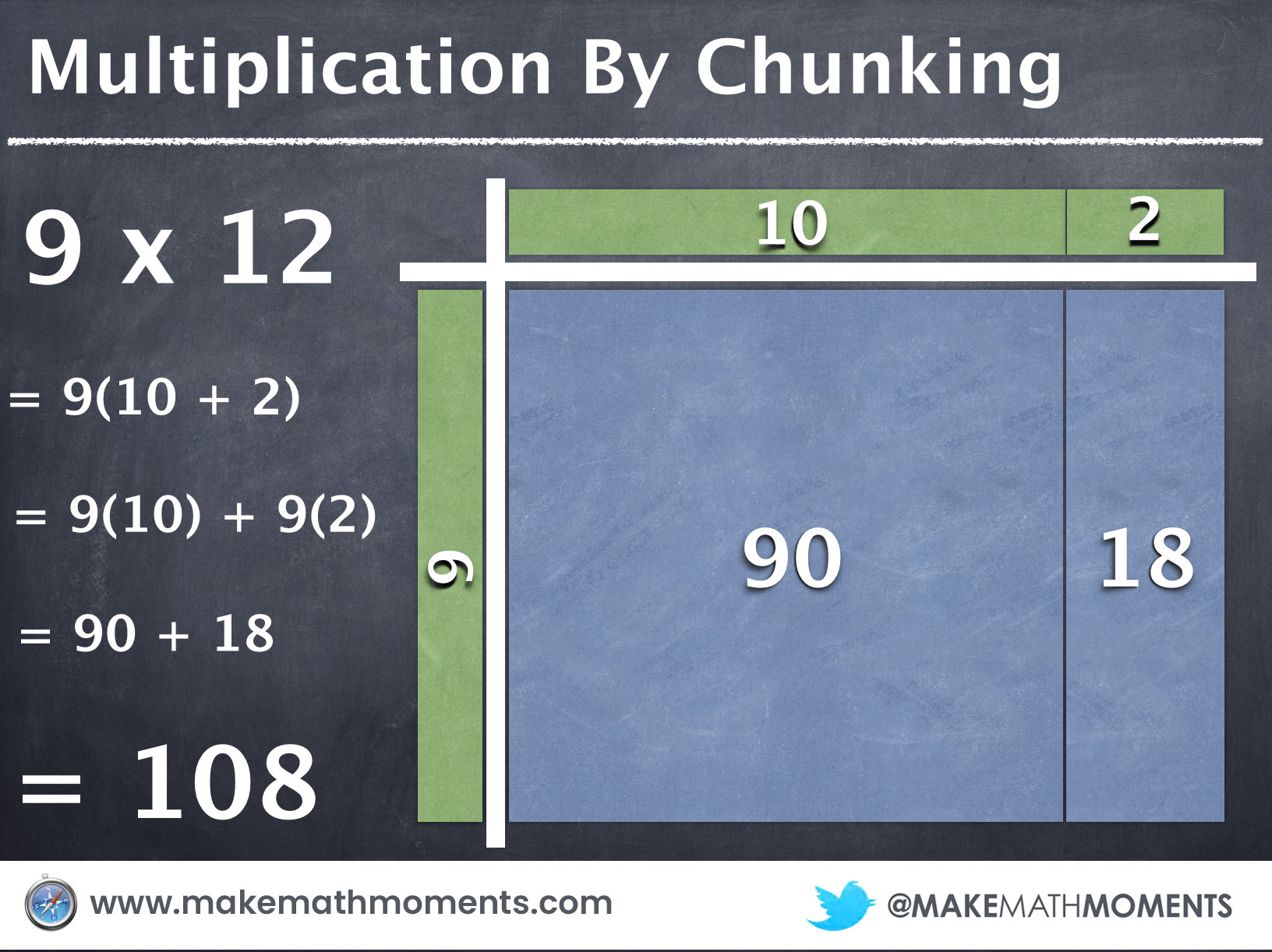If multiplication strategies are used consistently and with purpose, students can build their multiplication automaticity in order to create “friendly chunks”. The model above would suggest that this student may be comfortable multiplying by groups of 10 and thus can save a ton of work by chunking in such a manner until they feel comfortable with multiplying by groups of 12.

## Why It Is Important to Understand Multiplication

While I think there are huge advantages to having multiplication tables memorized, I think we need to be cautious about how we plan to get to that end goal. If we do not take time to clarify the how, we risk promoting the use of repetition through mass practice without promoting a deep conceptual understanding of what multiplication really represents. Even worse, promoting memorization without meaning could push students to dislike math and deter them from building a productive disposition towards the subject area we enjoy so much.

## Interested in more articles around memorizing multiplication tables?

Should We Stop Making Kids Memorize Times Tables? – Jo Boaler – US News